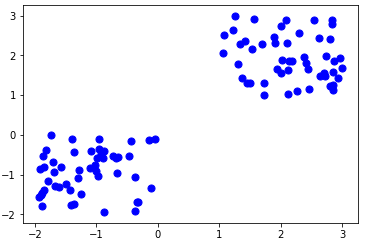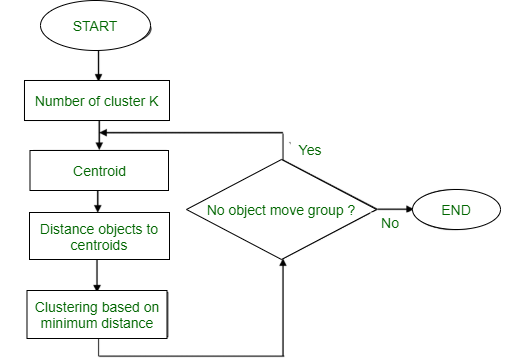Related Articles

# Partitioning Method (K-Mean) in Data Mining

• Last Updated : 05 Feb, 2020

Partitioning Method:
This clustering method classifies the information into multiple groups based on the characteristics and similarity of the data. Its the data analysts to specify the number of clusters that has to be generated for the clustering methods.

In the partitioning method when database(D) that contains multiple(N) objects then the partitioning method constructs user-specified(K) partitions of the data in which each partition represents a cluster and a particular region. There are many algorithms that come under partitioning method some of the popular ones are K-Mean, PAM(K-Mediods), CLARA algorithm (Clustering Large Applications) etc.

In this article, we will be seeing the working of K Mean algorithm in detail.

K-Mean (A centroid based Technique):
The K means algorithm takes the input parameter K from the user and partitions the dataset containing N objects into K clusters so that resulting similarity among the data objects inside the group (intracluster) is high but the similarity of data objects with the data objects from outside the cluster is low (intercluster). The similarity of the cluster is determined with respect to the mean value of the cluster.

It is a type of square error algorithm. At the start randomly k objects from the dataset are chosen in which each of the objects represents a cluster mean(centre). For the rest of the data objects, they are assigned to the nearest cluster based on their distance from the cluster mean. The new mean of each of the cluster is then calculated with the added data objects.

Algorithm: K mean:

```Input:
K: The number of clusters in which the dataset has to be divided
D: A dataset containing N number of objects

Output:
A dataset of K clusters ```

Method:

1. Randomly assign K objects from the dataset(D) as cluster centres(C)
2. (Re) Assign each object to which object is most similar based upon mean values.
3. Update Cluster means, i.e., Recalculate the mean of each cluster with the updated values.
4. Repeat Step 4 until no change occurs.Figure – K-mean Clustering

Flowchart:Figure – K-mean Clustering

Example: Suppose we want to group the visitors to a website using just their age as follows:

`16, 16, 17, 20, 20, 21, 21, 22, 23, 29, 36, 41, 42, 43, 44, 45, 61, 62, 66 `

Initial Cluster:

```K=2
Centroid(C1) = 16 
Centroid(C2) = 22  ```

Note: These two points are chosen randomly from the dataset.

Iteration-1:

```C1 = 16.33 [16, 16, 17]
C2 = 37.25 [20, 20, 21, 21, 22, 23, 29, 36, 41, 42, 43, 44, 45, 61, 62, 66] ```

Iteration-2:

```C1 = 19.55 [16, 16, 17, 20, 20, 21, 21, 22, 23]
C2 = 46.90 [29, 36, 41, 42, 43, 44, 45, 61, 62, 66] ```

Iteration-3:

```C1 = 20.50 [16, 16, 17, 20, 20, 21, 21, 22, 23, 29]
C2 = 48.89 [36, 41, 42, 43, 44, 45, 61, 62, 66] ```

Iteration-4:

```C1 = 20.50 [16, 16, 17, 20, 20, 21, 21, 22, 23, 29]
C2 = 48.89 [36, 41, 42, 43, 44, 45, 61, 62, 66] ```

No change Between Iteration 3 and 4, so we stop. Therefore we get the clusters (16-29) and (36-66) as 2 clusters we get using K Mean Algorithm.

Attention reader! Don’t stop learning now. Get hold of all the important CS Theory concepts for SDE interviews with the CS Theory Course at a student-friendly price and become industry ready.

My Personal Notes arrow_drop_up